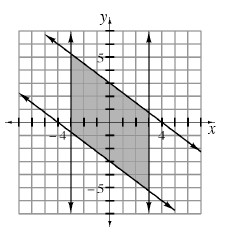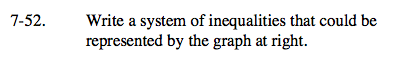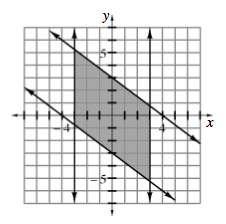Home > A2C > Chapter 7 > Lesson 7.1.4 > Problem7-52

7-52.

Write a system of inequalities that could be represented by the graph below. Homework Help ✎Start by finding an equation for each line, and then test points to determine which inequality to use.

$\text{One of the inequalities is: }y\leq -\frac{3}{4}x+3.$